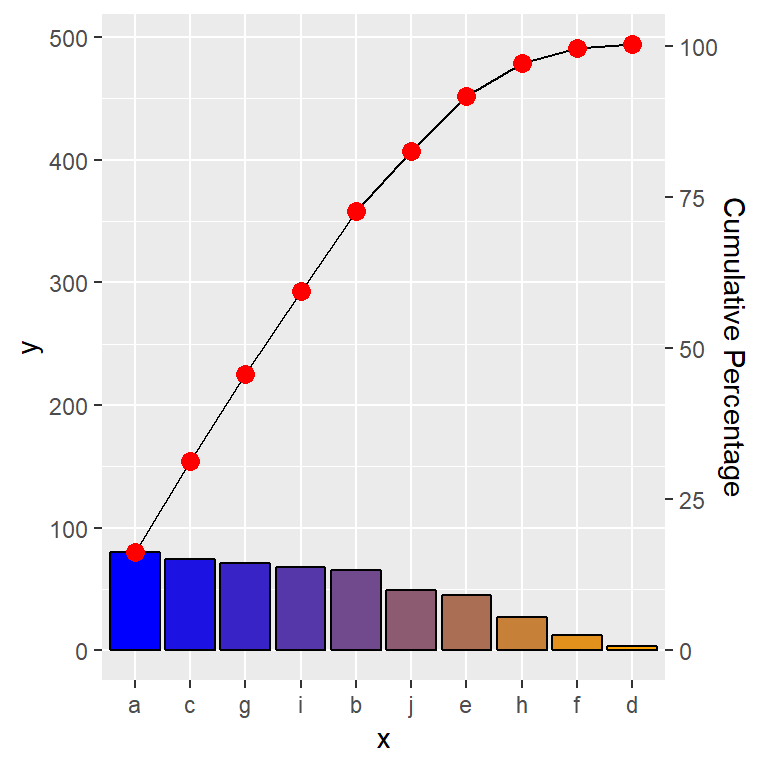# Description

ggplot stat function to produce a pareto chart.

# Usage

stat_pareto(mapping = NULL, data = NULL, geom = "point", position = "identity", show.legend = NA, inherit.aes = TRUE, group = 1, na.rm = FALSE, point.color = "black", point.size = 2, line.color = "black", line.size = 0.5, bars.fill = c("red", "white"), ...)

# Specialized Args:

group defines grouping for variable for pareto plot, default and suggested is 1.

point.color color, used to define point color of cumulative percentage line

point.size number, used to define point size of cumulative percentage line

line.color color, used to define line color of cumulative percentage line

line.size color, used to define line weight of cumulative percentage line

bars.fill character vector length 2, start and end colors for pareto bars.

# Examples

# Setup Data --------------------------------------------------------------
df <- data.frame(
x = letters[1:10],
y = as.integer(runif(n = 10, min = 0, max=100))
)
# Render Pareto Plot ------------------------------------------------------

ggplot(df, aes(x=x, y=y)) +
stat_pareto(point.color = "red",
point.size = 3,
line.color = "black",
#size.line = 1,
bars.fill = c("blue", "orange")
)